# Causal Analysis in Theory and Practice

## May 8, 2006

### Identifying conditional plans

Filed under: Book (J Pearl),Plans — moderator @ 12:00 am

Section 4.2 of the book (p. 113) gives an identification condition and estimation formula for the effect of a conditional action, namely, the effect of an action do(X=g(z)) where Z is a measurement taken prior to the action. Is this equation generalizable to the case of several actions, i.e., conditional plan?

The difficulty seen is that this formula was derived on the assumption that X does not change the value of Z. However, in a multi-action plan, some actions in X could change observations Z that are used to guide future actions. We do not have notation for distinguishing post-intevention from pre-intevention observations.

## 1 Comment »

1. The need for notational distinction between post-intevention from pre-inter-vention observations is valid, and will be satisfied in Chapter 7 where we deal with counterfactuals. The case of conditional plans, however, can be handled without resorting to richer notation. The reason is that the observations which dictate the choice of an action are not changed by that action, while those that have changed by previous actions are well captured by the P(y|do(x),z) notation.

To see that this is the case, however, we will need to introduce counterfactual notation, and then show how it can be eliminated from our expression. We will use bold letters to denote sets, and normal letters to denote individual elements. Also, capital letters will denote random variables, and small letters will denote possible values these variables could attain. We will write Yx to mean &#39;the value Y attains if we set variables X to values x.&#39; Similarly, YXg is taken to mean &#39;the value Y attains if we set variables X to whatever values they would have attained under the stochastic policy g.&#39; Note that Yx and YXg are both random variables, just as the original variable Y.

Say we have a set of K action variables X that occur in some temporal order. We will indicate the time at which a given variable is acted on by a superscript, so a variable X i occurs before X j if i &lt; j. For a given X i, we denote X&lt; i to be the set of action variables preceding X i.

We are interested in the probability distribution of a set of outcome variables Y, under a policy that sets the values of each X i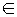X to the output of functions gi (known in advance) which pay attention to some set of prior variables Zi, as well as the previous interventions on X&lt; i. At the same time, the variables Zi are themselves affected by previous interventions. To define this recursion appropriately, we use an inductive definition. The base case is X1g = g1(Z1). The inductive case is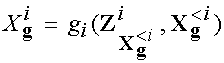. Here the subscript g represents the policy we use, in other words, g = {gi | i = 1, 2, …, K}. We can now write the quantity of interest: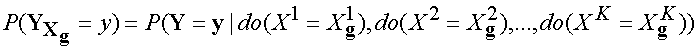Let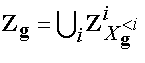. The key observation here is that if we observe Zg to take on particular values, Xg collapse to unique values as well because Xg is a function of Zg. We let xz = {x1z,…, xKz} be the values attained by Xg in the situation where Zg has been observed to equal z = {z1,…,zK}. We note here that if we know z, we can compute xz in advance, because the functions gi are fixed in advance and known to us. However, we don&#39;t know what values Zg might obtain, so we use case analysis to consider all possible value combinations. We then obtain:Here we note that Zi cannot depend on subsequent interventions. So we obtain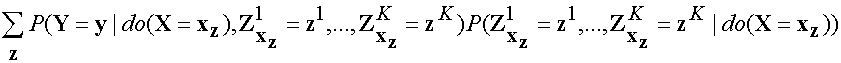Now we note that the subscripts in the first and second terms are redundant, since the do(xz) already implies such subscripts for all variables in the expression. Thus we can rewrite the target quantity as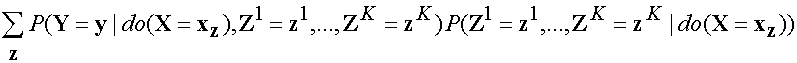or, more succinctly,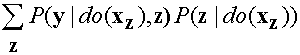We see that we can compute this expression from P(y | do(x)), z) and P(z | do(x)), where Y, X, Z are disjoint sets. Complete conditions for identifying these quantities from a joint distribution in a given graph G are given in , .

To summarize, though conditional plans are represented by complex nested counterfactual expressions, their identification can nevertheless be reduced to identification of conditional interventional distributions of the form P(y | do(x), z) (possibly with z being empty). Moreover, a complete condition for identifying such distributions from evidence exists.

Best wishes,
========Judea Pearl

References
 Shpitser, I., and Pearl, J. Identification of conditional interventional distributions. In Uncertainty in Artificial Intelligence (2006), vol. 22.
 Shpitser, I., and Pearl, J. Identification of joint interventional distributions in recursive semi-markovian causal models. In Twenty-First National Conference on Artificial Intelligence (2006).

Comment by Judea — May 8, 2006 @ 12:00 am